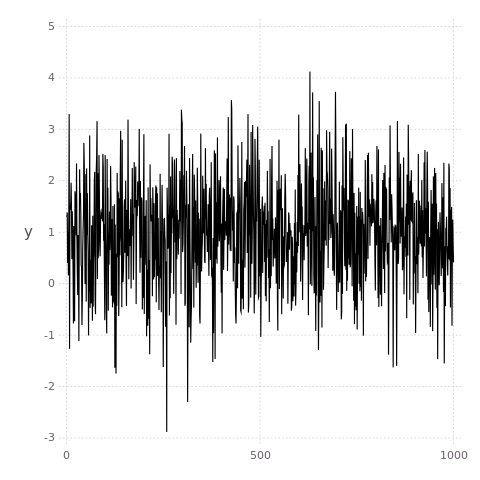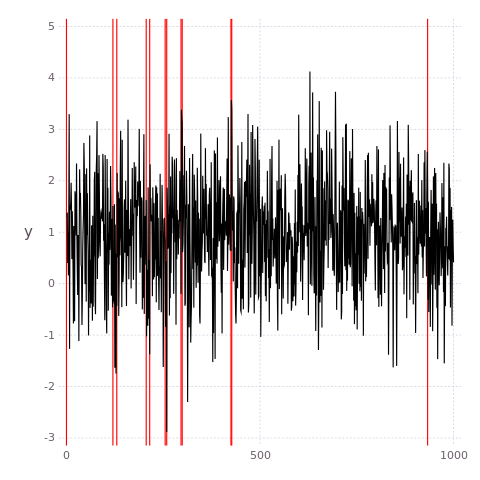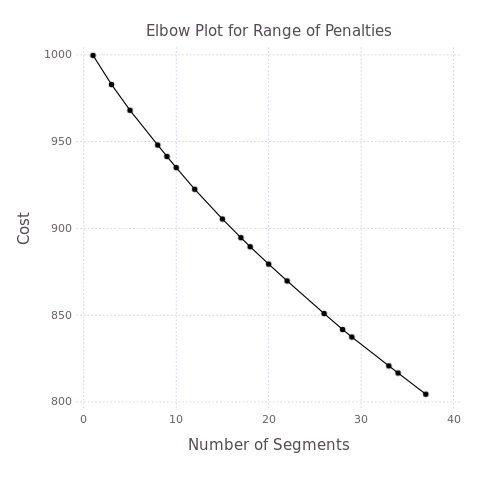16

5

10

4

# Changepoints.jlA Julia package for the detection of multiple changepoints in time series.

• Detection is based on optimising a cost function over segments of the data.
• Implementations of the most efficient search algorithms (PELT , Binary Segmentation).
• A wide choice of parametric cost functions already implemented such as a change in mean/variance/mean and variance for Normal errors.
• Changepoint algorithms have an interface which allows users to input their own cost functions

For a general overview of the multiple changepoint problem and mathematical details see PELT.

## Installation

Changepoints requires Julia version 0.7 or above. To install Changepoints simply run the following command inside Julia package mode (started by typing`]` in the Julia REPL):

``````(v0.7) pkg>  add Changepoints
``````

## Documentation

Most of the functionality of Changepoints has been documented. This is accessible in the Julia REPL in help mode. (started by typing '?' in the Julia REPL):

``````help?> @PELT
@PELT data changepoint_model [β₁ [β₂] ]

Runs the PELT algorithm on time series data using a specified changepoint_model and penalties.
If no penalty β₁ provided, a default of value log(length(data)) is used. If two penalties β₁ and β₂ are provided
then the CROPS algorithm is run which finds all optimal segmentations for all penalties between β₁ and β₂.

Example
≡≡≡≡≡≡≡≡≡

n = 1000
λ = 100
μ, σ = Normal(0.0, 10.0), 1.0
# Samples changepoints from Normal distribution with changing mean
sample, cps = @changepoint_sampler n λ Normal(μ, σ)
# Run PELT on sample
pelt_cps, pelt_cost = @PELT sample Normal(:?, σ)
``````

# Usage

As an example first we simulate a time series with multiple changes in mean and then segment it using PELT plotting the time series as we go.

## Simulation

This code simulates a time series of length n with segments that have lengths drawn from a Poisson distribution with mean lambda. The variance is fixed in this case as one but for each new segment a new mean is drawn from a standard gaussian distribution.

``````n = 1000                   # Sample size
λ = 70                     # freq of changepoints
μ, σ = Normal(0,1), 1.0
data, cps = @changepoint_sampler n λ Normal(μ, σ)
``````To segment the data assuming it is Normally distributed and has a constant variance of one, using a default penalty (the log of the length of the data) can be done using the @PELT macro. Currently, this package supports the Gadfly and Winston packages for the convenient plotting of the results. These packages must be explicity loaded to make use of this functionality. If the plotting package was loaded after Changepoints, then the user must run an additional command to load the plotting functionaly, e.g. `Changepoints.Gadfly_init()`.

``````pelt_cps, cost = @PELT data Normal(:?, 1.0)
plot(data, pelt_cps)
``````## Penalty selection

The methods implemented view the problem as one of optimising a penalised cost function where the penalty comes in whenever a new changepoint is added. Assuming we have specified the correct parametric (non-parametric cost coming soon) model/cost function then the only area of possible misspecification is in the value of the penalty. There is no "correct" choice of penalty however it can be very instructive to look at the segmentations and especially the number of changepoints for a range of penalties. The Changepoints for a Range Of Penalties (CROPS) method allows us to do this efficiently using PELT, by exploiting the relationship between the penalised and constrained versions of the same optimisation problem. For more information see CROPS.

To run the PELT algorithm for a range of penalties say pen1 to pen2 where pen1 < pen2 then we can use the following code:

``````crops_output = @PELT data Normal(:?, 1.0) pen1 pen2
``````

Having segmented the dataset for a range of penalties the problem now becomes one of model selection. Again, if a plotting package has been loaded, we can create a so called "elbow" plot from these results.

``````plot(crops_output)
``````03/06/2015

2 months ago

186 commits